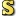﻿Multiply Method
Cove - A Practical Quantum Computer Programming Framework
Multiply Method
NamespacesCove.ClassicalUtilitiesComplexMatrixMultiply()()()Members
IconMemberDescriptionMultiply(ComplexMatrix, ComplexMatrix)
Perform matrix multiplicationMultiply(Double, ComplexMatrix)
Multiple a matrix by the given value

Assembly: Cove.ClassicalUtilities (Module: Cove.ClassicalUtilities) Version: 1.0.0.0 (1.0.0.0)